# Selina Solutions Concise Mathematics Class 6 Chapter 19 Fundamental Operations Exercise 19(B)

Selina Solutions Concise Mathematics Class 6 Chapter 19 Fundamental Operations Exercise 19(B) provides answers based on sum and evaluation of algebraic expressions. The solutions offer shortcut tricks to solve the problems with ease. Students analyse the type of problems that would appear in the annual exam, using solutions PDF on a daily basis. The PDFs of solutions can be used by the students in clearing their doubts that appear while solving. Here, students can find Selina Solutions Concise Mathematics Class 6 Chapter 19 Fundamental Operations Exercise 19(B) free PDF, from the links given below.

## Selina Solutions Concise Mathematics Class 6 Chapter 19: Fundamental Operations Exercise 19(B) Download PDF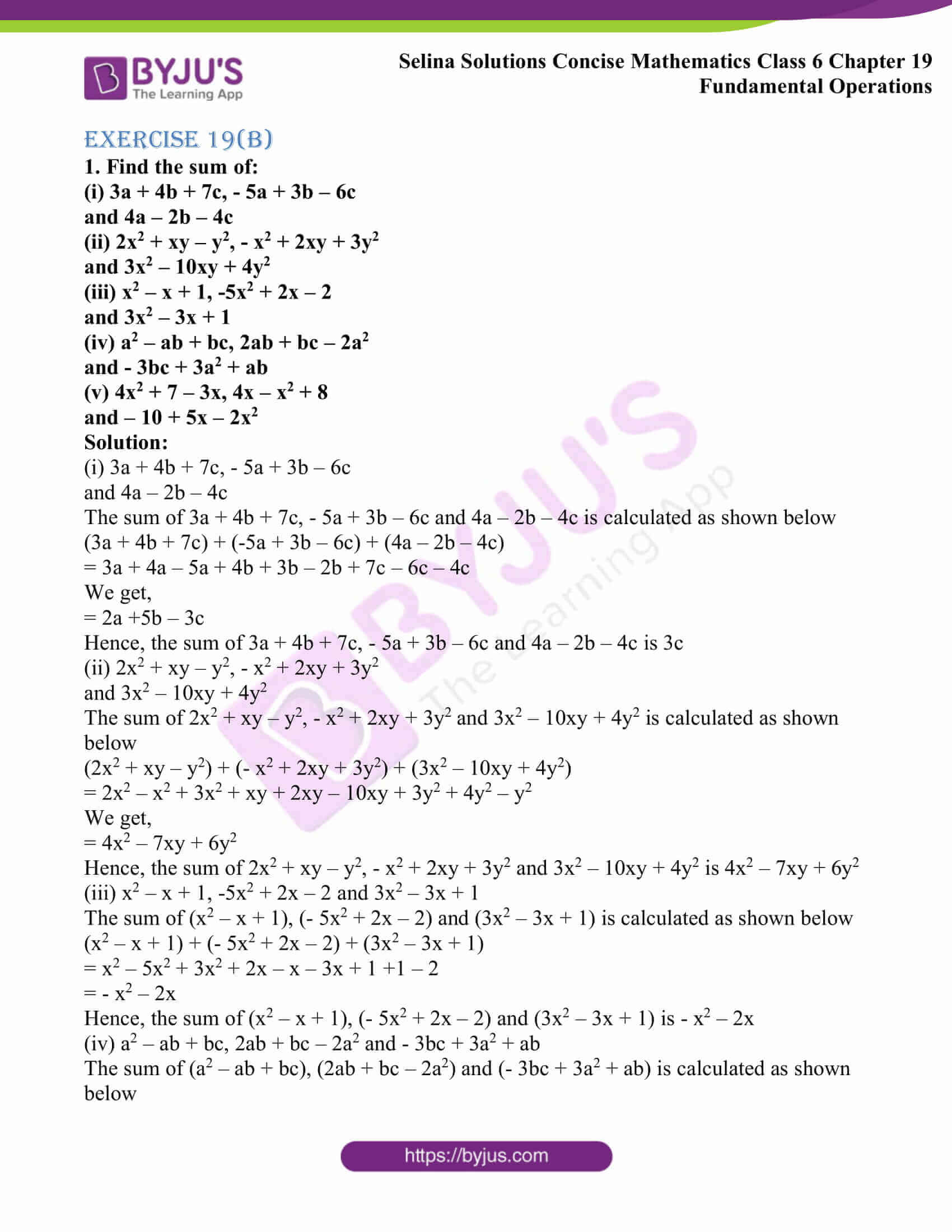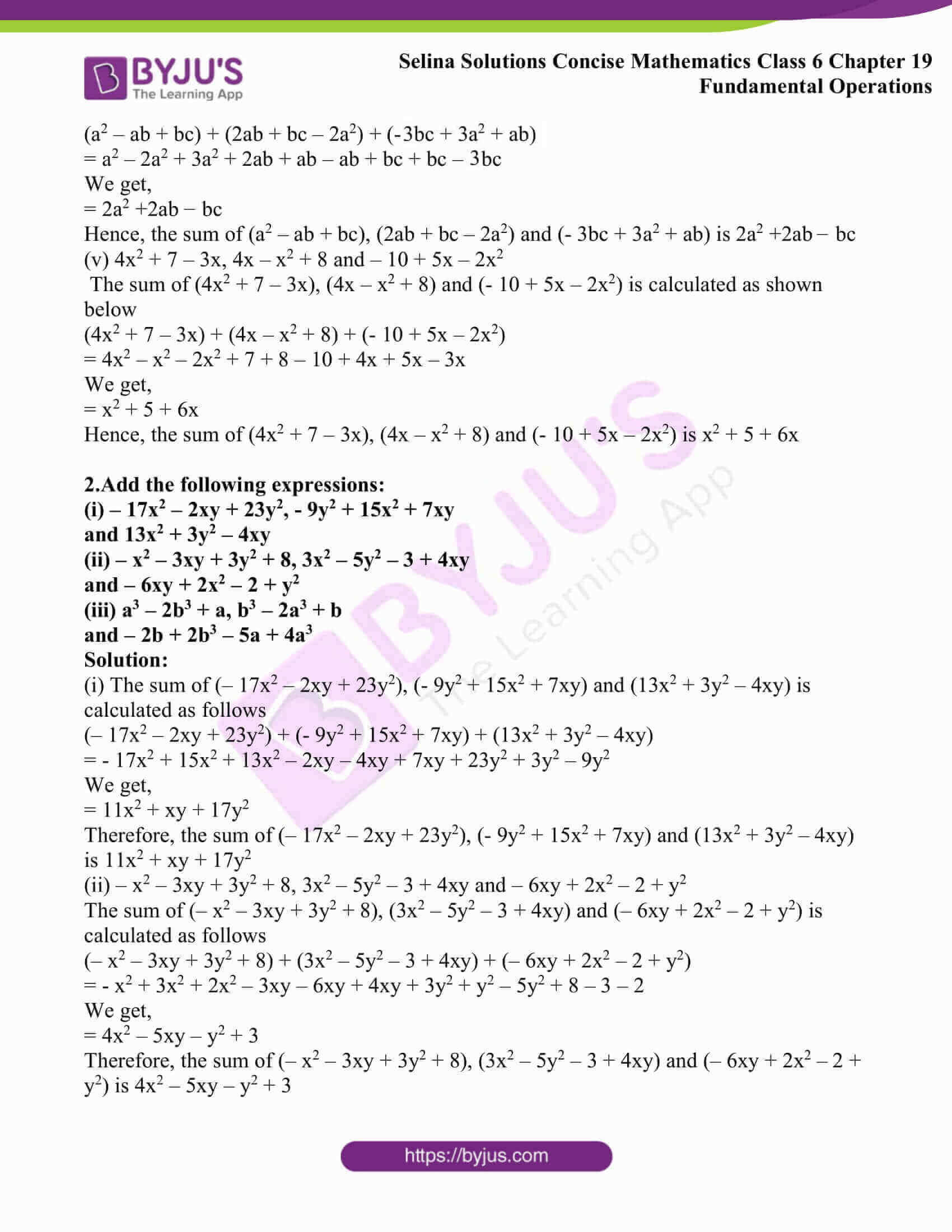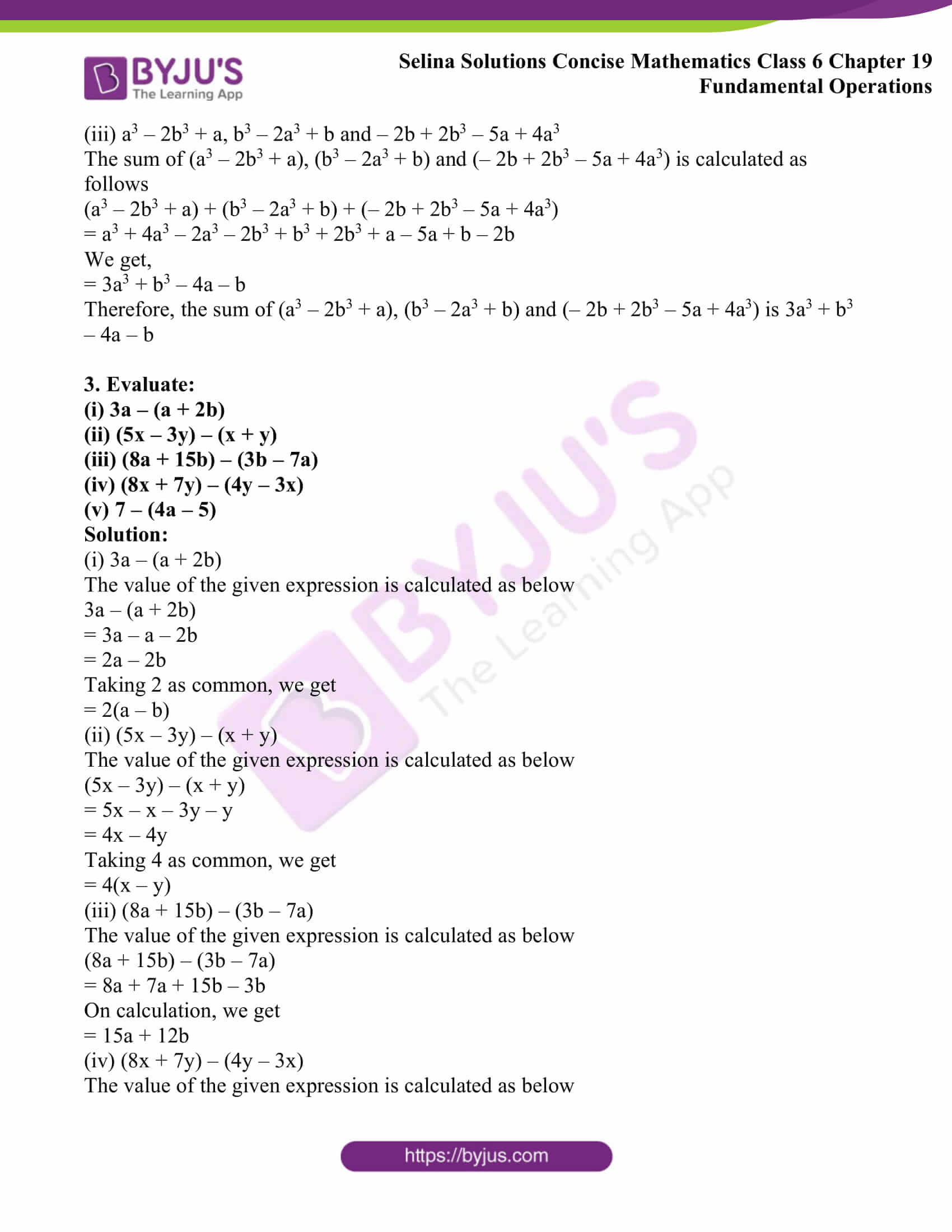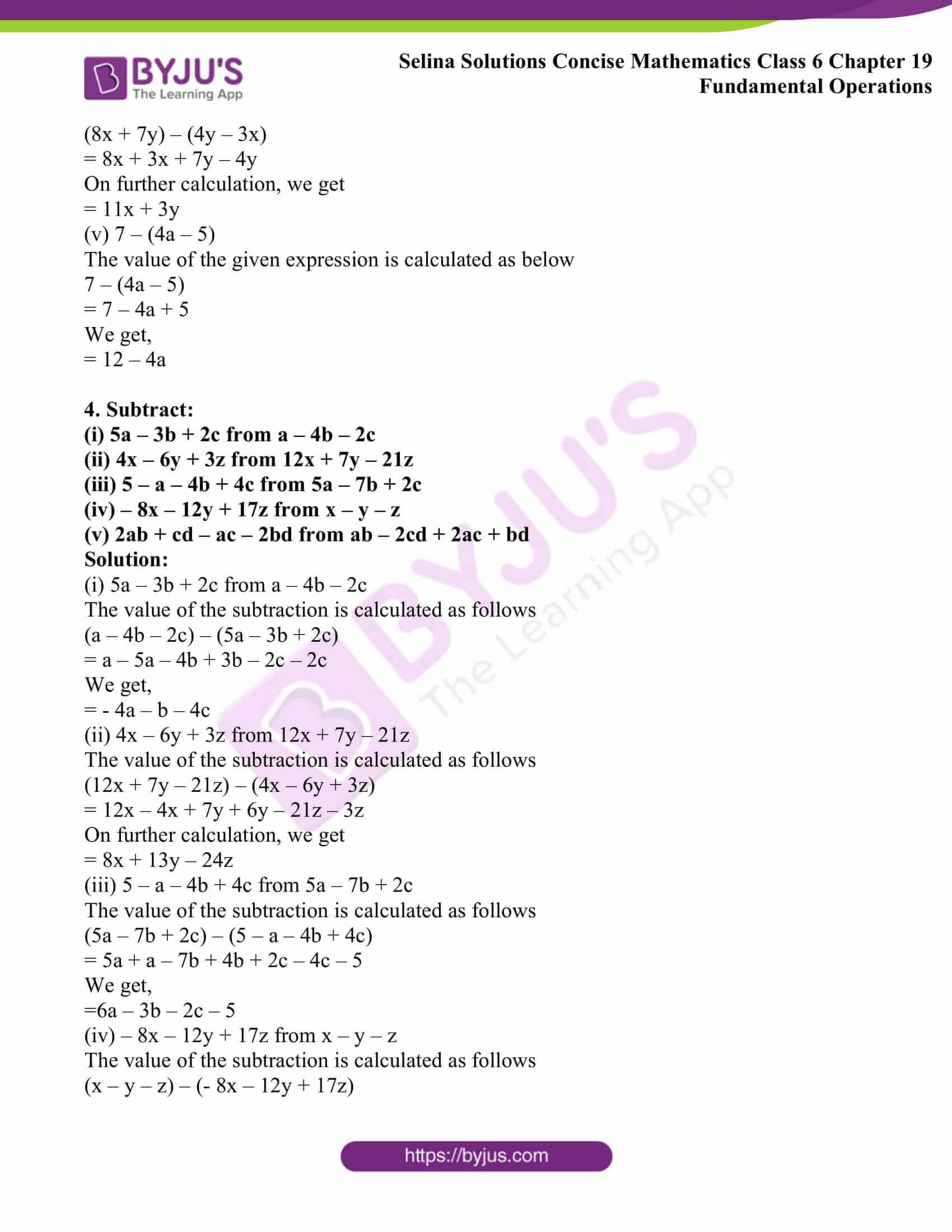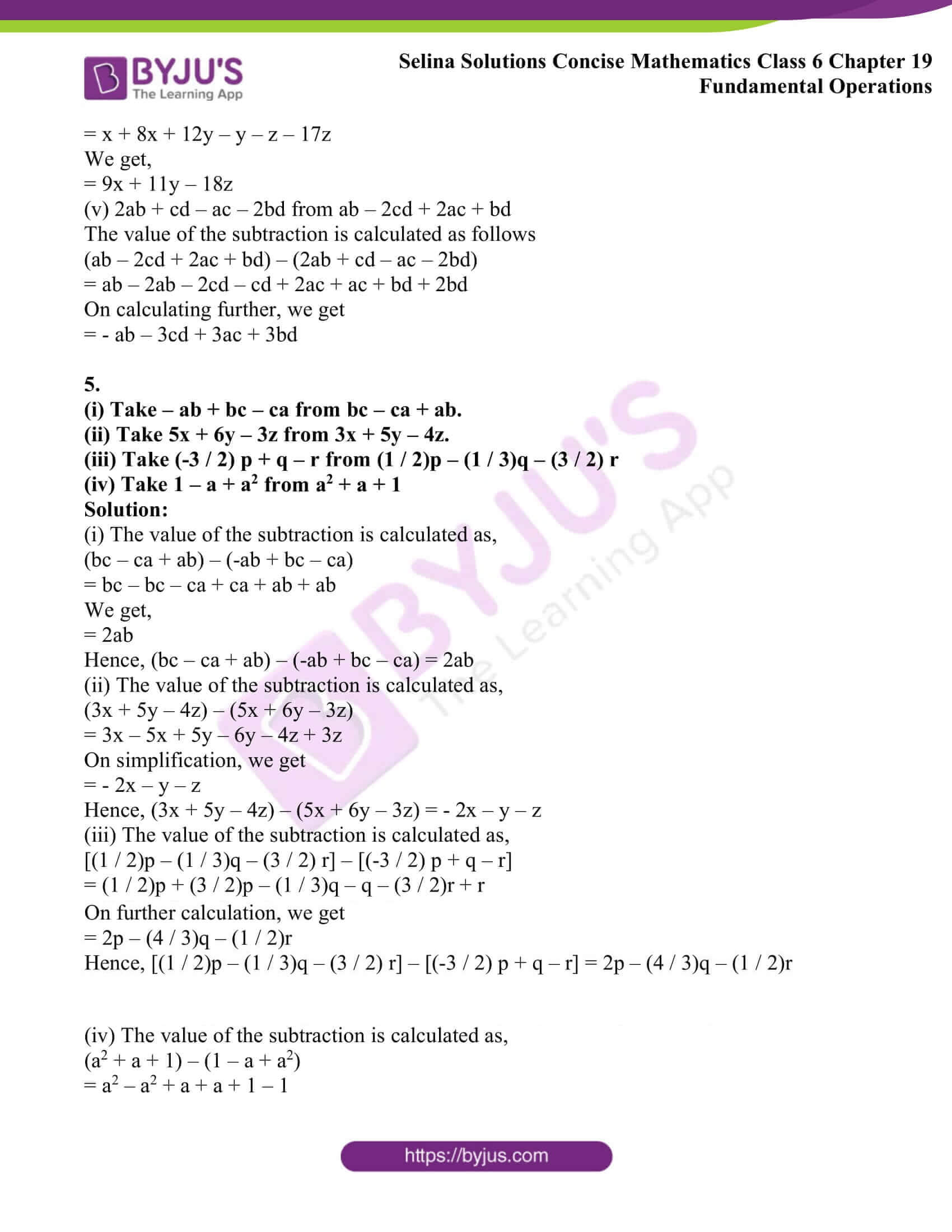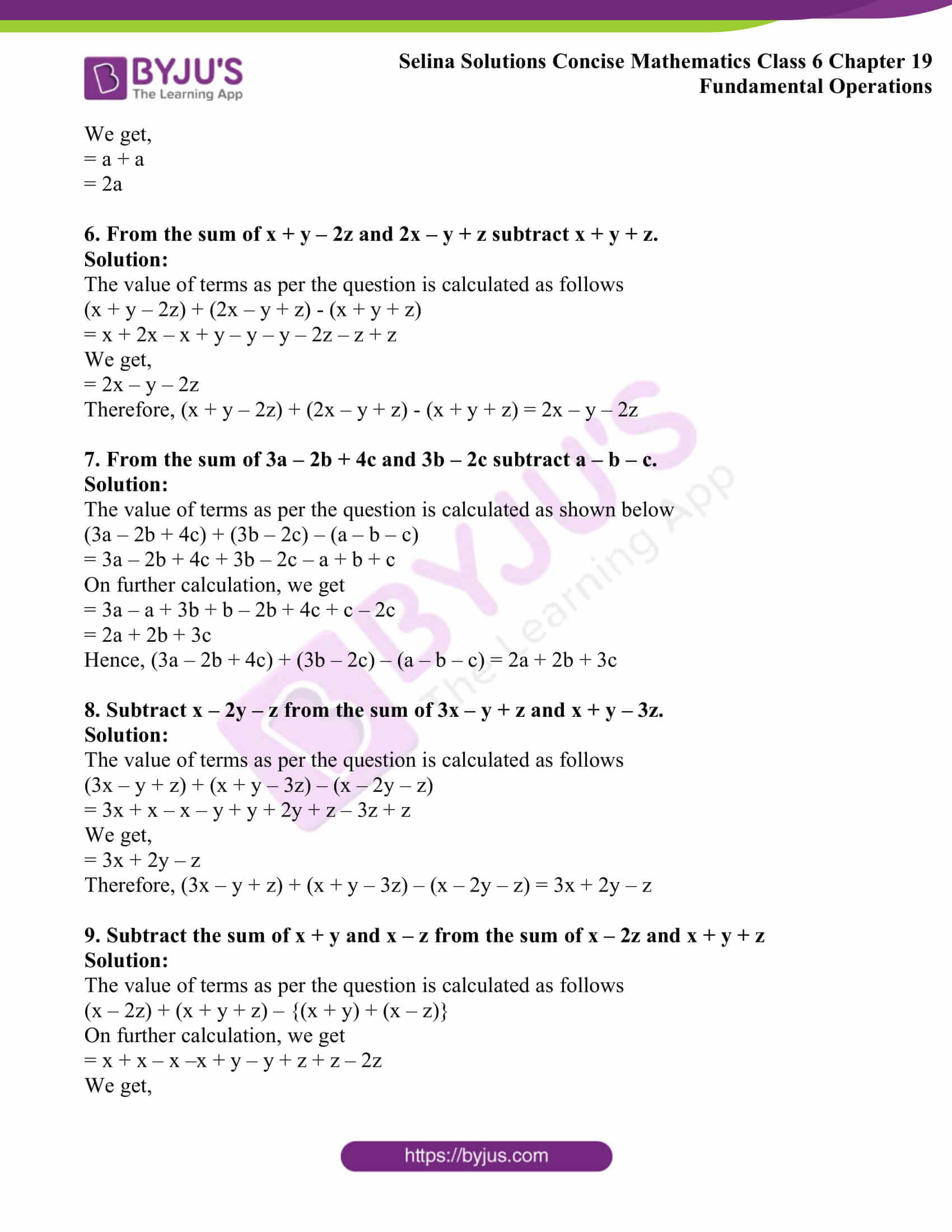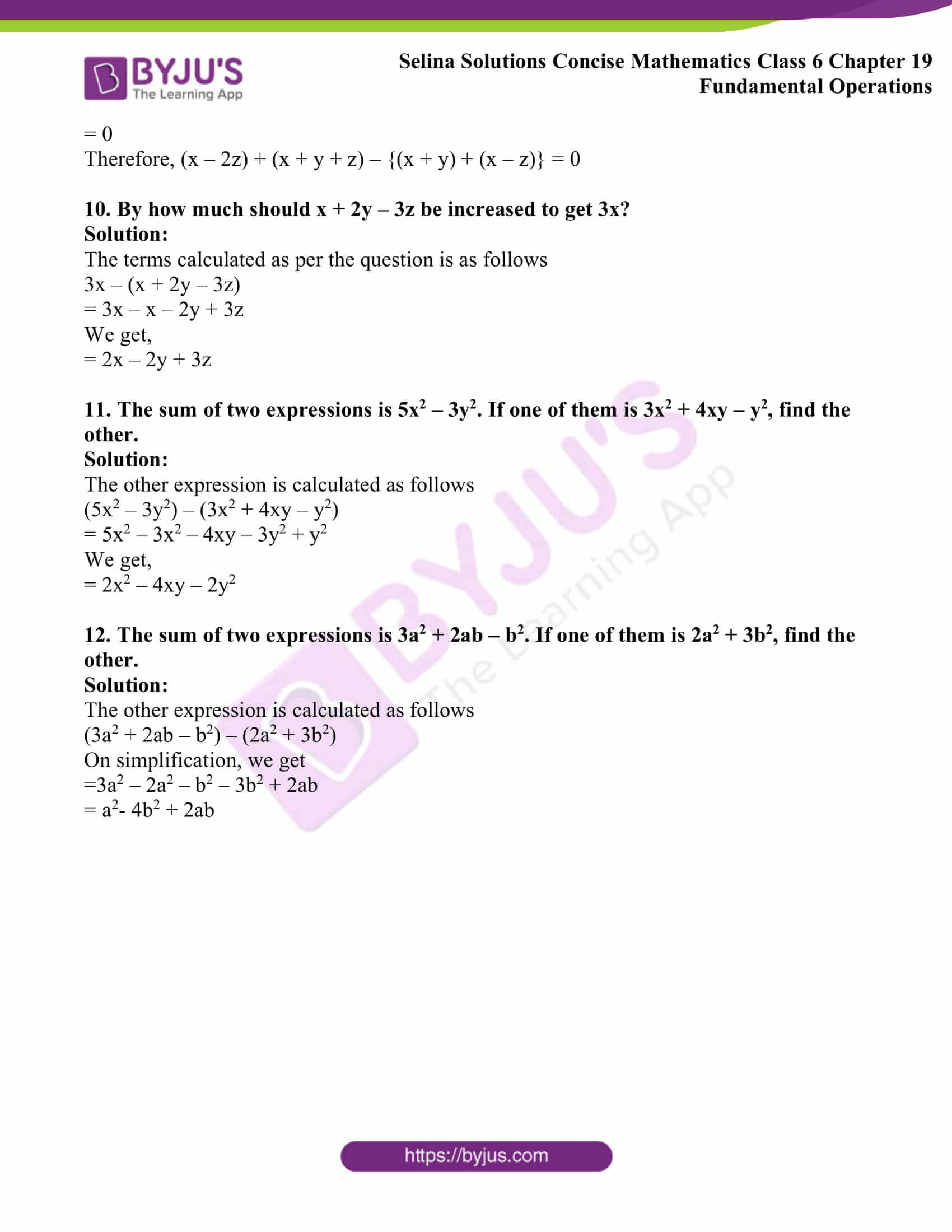### Access other exercises of Selina Solutions Concise Mathematics Class 6 Chapter 19: Fundamental Operations

Exercise 19(A) Solutions

Exercise 19(C) Solutions

Exercise 19(D) Solutions

### Access Selina Solutions Concise Mathematics Class 6 Chapter 19: Fundamental Operations Exercise 19(B)

Exercise 19(B)

1. Find the sum of:

(i) 3a + 4b + 7c, – 5a + 3b – 6c

and 4a – 2b – 4c

(ii) 2x2 + xy – y2, – x2 + 2xy + 3y2

and 3x2 – 10xy + 4y2

(iii) x2 – x + 1, -5x2 + 2x – 2

and 3x2 – 3x + 1

(iv) a2 – ab + bc, 2ab + bc – 2a2

and – 3bc + 3a2 + ab

(v) 4x2 + 7 – 3x, 4x – x2 + 8

and – 10 + 5x – 2x2

Solution:

(i) 3a + 4b + 7c, – 5a + 3b – 6c

and 4a – 2b – 4c

The sum of 3a + 4b + 7c, – 5a + 3b – 6c and 4a – 2b – 4c is calculated as shown below

(3a + 4b + 7c) + (-5a + 3b – 6c) + (4a – 2b – 4c)

= 3a + 4a – 5a + 4b + 3b – 2b + 7c – 6c – 4c

We get,

= 2a +5b – 3c

Hence, the sum of 3a + 4b + 7c, – 5a + 3b – 6c and 4a – 2b – 4c is 3c

(ii) 2x2 + xy – y2, – x2 + 2xy + 3y2

and 3x2 – 10xy + 4y2

The sum of 2x2 + xy – y2, – x2 + 2xy + 3y2 and 3x2 – 10xy + 4y2 is calculated as shown below

(2x2 + xy – y2) + (- x2 + 2xy + 3y2) + (3x2 – 10xy + 4y2)

= 2x2 – x2 + 3x2 + xy + 2xy – 10xy + 3y2 + 4y2 – y2

We get,

= 4x2 – 7xy + 6y2

Hence, the sum of 2x2 + xy – y2, – x2 + 2xy + 3y2 and 3x2 – 10xy + 4y2 is 4x2 – 7xy + 6y2

(iii) x2 – x + 1, -5x2 + 2x – 2 and 3x2 – 3x + 1

The sum of (x2 – x + 1), (- 5x2 + 2x – 2) and (3x2 – 3x + 1) is calculated as shown below

(x2 – x + 1) + (- 5x2 + 2x – 2) + (3x2 – 3x + 1)

= x2 – 5x2 + 3x2 + 2x – x – 3x + 1 +1 – 2

= – x2 – 2x

Hence, the sum of (x2 – x + 1), (- 5x2 + 2x – 2) and (3x2 – 3x + 1) is – x2 – 2x

(iv) a2 – ab + bc, 2ab + bc – 2a2 and – 3bc + 3a2 + ab

The sum of (a2 – ab + bc), (2ab + bc – 2a2) and (- 3bc + 3a2 + ab) is calculated as shown below

(a2 – ab + bc) + (2ab + bc – 2a2) + (- 3bc + 3a2 + ab)

= a2 – 2a2 + 3a2 + 2ab + ab – ab + bc + bc – 3bc

We get,

= 2a2 +2ab – bc

Hence, the sum of (a2 – ab + bc), (2ab + bc – 2a2) and (- 3bc + 3a2 + ab) is 2a2 +2ab – bc

(v) 4x2 + 7 – 3x, 4x – x2 + 8 and – 10 + 5x – 2x2

The sum of (4x2 + 7 – 3x), (4x – x2 + 8) and (- 10 + 5x – 2x2) is calculated as shown below

(4x2 + 7 – 3x) + (4x – x2 + 8) + (- 10 + 5x – 2x2)

= 4x2 – x2 – 2x2 + 7 + 8 – 10 + 4x + 5x – 3x

We get,

= x2 + 5 + 6x

Hence, the sum of (4x2 + 7 – 3x), (4x – x2 + 8) and (- 10 + 5x – 2x2) is x2 + 5 + 6x

(i) – 17x2 – 2xy + 23y2, – 9y2 + 15x2 + 7xy

and 13x2 + 3y2 – 4xy

(ii) – x2 – 3xy + 3y2 + 8, 3x2 – 5y2 – 3 + 4xy

and – 6xy + 2x2 – 2 + y2

(iii) a3 – 2b3 + a, b3 – 2a3 + b

and – 2b + 2b3 – 5a + 4a3

Solution:

(i) The sum of (– 17x2 – 2xy + 23y2), (- 9y2 + 15x2 + 7xy) and (13x2 + 3y2 – 4xy) is calculated as follows

(– 17x2 – 2xy + 23y2) + (- 9y2 + 15x2 + 7xy) + (13x2 + 3y2 – 4xy)

= – 17x2 + 15x2 + 13x2 – 2xy – 4xy + 7xy + 23y2 + 3y2 – 9y2

We get,

= 11x2 + xy + 17y2

Therefore, the sum of (– 17x2 – 2xy + 23y2), (- 9y2 + 15x2 + 7xy) and (13x2 + 3y2 – 4xy) is 11x2 + xy + 17y2

(ii) – x2 – 3xy + 3y2 + 8, 3x2 – 5y2 – 3 + 4xy and – 6xy + 2x2 – 2 + y2

The sum of (– x2 – 3xy + 3y2 + 8), (3x2 – 5y2 – 3 + 4xy) and (– 6xy + 2x2 – 2 + y2) is calculated as follows

(– x2 – 3xy + 3y2 + 8) + (3x2 – 5y2 – 3 + 4xy) + (– 6xy + 2x2 – 2 + y2)

= – x2 + 3x2 + 2x2 – 3xy – 6xy + 4xy + 3y2 + y2 – 5y2 + 8 – 3 – 2

We get,

= 4x2 – 5xy – y2 + 3

Therefore, the sum of (– x2 – 3xy + 3y2 + 8), (3x2 – 5y2 – 3 + 4xy) and (– 6xy + 2x2 – 2 + y2) is 4x2 – 5xy – y2 + 3

(iii) a3 – 2b3 + a, b3 – 2a3 + b and – 2b + 2b3 – 5a + 4a3

The sum of (a3 – 2b3 + a), (b3 – 2a3 + b) and (– 2b + 2b3 – 5a + 4a3) is calculated as follows

(a3 – 2b3 + a) + (b3 – 2a3 + b) + (– 2b + 2b3 – 5a + 4a3)

= a3 + 4a3 – 2a3 – 2b3 + b3 + 2b3 + a – 5a + b – 2b

We get,

= 3a3 + b3 – 4a – b

Therefore, the sum of (a3 – 2b3 + a), (b3 – 2a3 + b) and (– 2b + 2b3 – 5a + 4a3) is 3a3 + b3 – 4a – b

3. Evaluate:

(i) 3a – (a + 2b)

(ii) (5x – 3y) – (x + y)

(iii) (8a + 15b) – (3b – 7a)

(iv) (8x + 7y) – (4y – 3x)

(v) 7 – (4a – 5)

Solution:

(i) 3a – (a + 2b)

The value of the given expression is calculated as below

3a – (a + 2b)

= 3a – a – 2b

= 2a – 2b

Taking 2 as common, we get

= 2(a – b)

(ii) (5x – 3y) – (x + y)

The value of the given expression is calculated as below

(5x – 3y) – (x + y)

= 5x – x – 3y – y

= 4x – 4y

Taking 4 as common, we get

= 4(x – y)

(iii) (8a + 15b) – (3b – 7a)

The value of the given expression is calculated as below

(8a + 15b) – (3b – 7a)

= 8a + 7a + 15b – 3b

On calculation, we get

= 15a + 12b

(iv) (8x + 7y) – (4y – 3x)

The value of the given expression is calculated as below

(8x + 7y) – (4y – 3x)

= 8x + 3x + 7y – 4y

On further calculation, we get

= 11x + 3y

(v) 7 – (4a – 5)

The value of the given expression is calculated as below

7 – (4a – 5)

= 7 – 4a + 5

We get,

= 12 – 4a

4. Subtract:

(i) 5a – 3b + 2c from a – 4b – 2c

(ii) 4x – 6y + 3z from 12x + 7y – 21z

(iii) 5 – a – 4b + 4c from 5a – 7b + 2c

(iv) – 8x – 12y + 17z from x – y – z

(v) 2ab + cd – ac – 2bd from ab – 2cd + 2ac + bd

Solution:

(i) 5a – 3b + 2c from a – 4b – 2c

The value of the subtraction is calculated as follows

(a – 4b – 2c) – (5a – 3b + 2c)

= a – 5a – 4b + 3b – 2c – 2c

We get,

= – 4a – b – 4c

(ii) 4x – 6y + 3z from 12x + 7y – 21z

The value of the subtraction is calculated as follows

(12x + 7y – 21z) – (4x – 6y + 3z)

= 12x – 4x + 7y + 6y – 21z – 3z

On further calculation, we get

= 8x + 13y – 24z

(iii) 5 – a – 4b + 4c from 5a – 7b + 2c

The value of the subtraction is calculated as follows

(5a – 7b + 2c) – (5 – a – 4b + 4c)

= 5a + a – 7b + 4b + 2c – 4c – 5

We get,

=6a – 3b – 2c – 5

(iv) – 8x – 12y + 17z from x – y – z

The value of the subtraction is calculated as follows

(x – y – z) – (- 8x – 12y + 17z)

= x + 8x + 12y – y – z – 17z

We get,

= 9x + 11y – 18z

(v) 2ab + cd – ac – 2bd from ab – 2cd + 2ac + bd

The value of the subtraction is calculated as follows

(ab – 2cd + 2ac + bd) – (2ab + cd – ac – 2bd)

= ab – 2ab – 2cd – cd + 2ac + ac + bd + 2bd

On calculating further, we get

= – ab – 3cd + 3ac + 3bd

5.

(i) Take – ab + bc – ca from bc – ca + ab.

(ii) Take 5x + 6y – 3z from 3x + 5y – 4z.

(iii) Take (-3 / 2) p + q – r from (1 / 2)p – (1 / 3)q – (3 / 2) r

(iv) Take 1 – a + a2 from a2 + a + 1

Solution:

(i) The value of the subtraction is calculated as,

(bc – ca + ab) – (-ab + bc – ca)

= bc – bc – ca + ca + ab + ab

We get,

= 2ab

Hence, (bc – ca + ab) – (-ab + bc – ca) = 2ab

(ii) The value of the subtraction is calculated as,

(3x + 5y – 4z) – (5x + 6y – 3z)

= 3x – 5x + 5y – 6y – 4z + 3z

On simplification, we get

= – 2x – y – z

Hence, (3x + 5y – 4z) – (5x + 6y – 3z) = – 2x – y – z

(iii) The value of the subtraction is calculated as,

[(1 / 2)p – (1 / 3)q – (3 / 2) r] – [(-3 / 2) p + q – r]

= (1 / 2)p + (3 / 2)p – (1 / 3)q – q – (3 / 2)r + r

On further calculation, we get

= 2p – (4 / 3)q – (1 / 2)r

Hence, [(1 / 2)p – (1 / 3)q – (3 / 2) r] – [(-3 / 2) p + q – r] = 2p – (4 / 3)q – (1 / 2)r

(iv) The value of the subtraction is calculated as,

(a2 + a + 1) – (1 – a + a2)

= a2 – a2 + a + a + 1 – 1

We get,

= a + a

= 2a

6. From the sum of x + y – 2z and 2x – y + z subtract x + y + z.

Solution:

The value of terms as per the question is calculated as follows

(x + y – 2z) + (2x – y + z) – (x + y + z)

= x + 2x – x + y – y – y – 2z – z + z

We get,

= 2x – y – 2z

Therefore, (x + y – 2z) + (2x – y + z) – (x + y + z) = 2x – y – 2z

7. From the sum of 3a – 2b + 4c and 3b – 2c subtract a – b – c.

Solution:

The value of terms as per the question is calculated as shown below

(3a – 2b + 4c) + (3b – 2c) – (a – b – c)

= 3a – 2b + 4c + 3b – 2c – a + b + c

On further calculation, we get

= 3a – a + 3b + b – 2b + 4c + c – 2c

= 2a + 2b + 3c

Hence, (3a – 2b + 4c) + (3b – 2c) – (a – b – c) = 2a + 2b + 3c

8. Subtract x – 2y – z from the sum of 3x – y + z and x + y – 3z.

Solution:

The value of terms as per the question is calculated as follows

(3x – y + z) + (x + y – 3z) – (x – 2y – z)

= 3x + x – x – y + y + 2y + z – 3z + z

We get,

= 3x + 2y – z

Therefore, (3x – y + z) + (x + y – 3z) – (x – 2y – z) = 3x + 2y – z

9. Subtract the sum of x + y and x – z from the sum of x – 2z and x + y + z

Solution:

The value of terms as per the question is calculated as follows

(x – 2z) + (x + y + z) – {(x + y) + (x – z)}

On further calculation, we get

= x + x – x –x + y – y + z + z – 2z

We get,

= 0

Therefore, (x – 2z) + (x + y + z) – {(x + y) + (x – z)} = 0

10. By how much should x + 2y – 3z be increased to get 3x?

Solution:

The terms calculated as per the question is as follows

3x – (x + 2y – 3z)

= 3x – x – 2y + 3z

We get,

= 2x – 2y + 3z

11. The sum of two expressions is 5x2 – 3y2. If one of them is 3x2 + 4xy – y2, find the other.

Solution:

The other expression is calculated as follows

(5x2 – 3y2) – (3x2 + 4xy – y2)

= 5x2 – 3x2 – 4xy – 3y2 + y2

We get,

= 2x2 – 4xy – 2y2

12. The sum of two expressions is 3a2 + 2ab – b2. If one of them is 2a2 + 3b2, find the other.

Solution:

The other expression is calculated as follows

(3a2 + 2ab – b2) – (2a2 + 3b2)

On simplification, we get

=3a2 – 2a2 – b2 – 3b2 + 2ab

= a2– 4b2 + 2ab